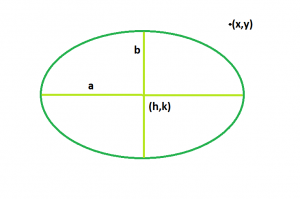# Check if a point is inside, outside or on the ellipse

Given an ellipse centered at (h, k), with semi-major axis a, semi-minor axis b, both aligned with the Cartesian plane. The task is to determine if the point (x, y) is within the area bounded by the ellipse.

Examples:

```Input: h = 0, k = 0, x = 2, y = 1, a = 4, b = 5
Output: Inside

Input: h = 1, k = 2, x = 200, y = 100, a = 6, b = 5
Output: Outside
```

## Recommended: Please try your approach on {IDE} first, before moving on to the solution.Approach: We have to solve the equation of ellipse for the given point (x, y),

(x-h)^2/a^2 + (y-k)^2/b^2 <= 1

If in the inequation, results comes less than 1 then the point lies within, else if it comes exact 1 then the point lies on the ellipse, and if the inequation is unsatisfied then point lies outside of the ellipse.

Below is the implementation of the above approach:

## C++

 `// C++ Program to check if the point ` `// lies within the ellipse or not ` `#include ` `using` `namespace` `std; ` ` `  `// Function to check the point ` `int` `checkpoint(``int` `h, ``int` `k, ``int` `x, ``int` `y, ``int` `a, ``int` `b) ` `{ ` ` `  `    ``// checking the equation of ` `    ``// ellipse with the given point ` `    ``int` `p = (``pow``((x - h), 2) / ``pow``(a, 2)) ` `            ``+ (``pow``((y - k), 2) / ``pow``(b, 2)); ` ` `  `    ``return` `p; ` `} ` ` `  `// Driver code ` `int` `main() ` `{ ` `    ``int` `h = 0, k = 0, x = 2, y = 1, a = 4, b = 5; ` ` `  `    ``if` `(checkpoint(h, k, x, y, a, b) > 1) ` `        ``cout << ``"Outside"` `<< endl; ` ` `  `    ``else` `if` `(checkpoint(h, k, x, y, a, b) == 1) ` `        ``cout << ``"On the ellipse"` `<< endl; ` ` `  `    ``else` `        ``cout << ``"Inside"` `<< endl; ` ` `  `    ``return` `0; ` `} `

## Java

 `// Java Program to check if the point ` `// lies within the ellipse or not ` `import` `java.util.*; ` ` `  `class` `solution ` `{ ` `  `  `// Function to check the point ` `static` `int` `checkpoint(``int` `h, ``int` `k, ``int` `x, ``int` `y, ``int` `a, ``int` `b) ` `{ ` ` `  `    ``// checking the equation of ` `    ``// ellipse with the given point ` `    ``int` `p = ((``int``)Math.pow((x - h), ``2``) / (``int``)Math.pow(a, ``2``)) ` `            ``+ ((``int``)Math.pow((y - k), ``2``) / (``int``)Math.pow(b, ``2``)); ` ` `  `    ``return` `p; ` `} ` ` `  `//Driver code ` `public` `static` `void` `main(String arr[]) ` `{ ` `   `  `    ``int` `h = ``0``, k = ``0``, x = ``2``, y = ``1``, a = ``4``, b = ``5``; ` ` `  `    ``if` `(checkpoint(h, k, x, y, a, b) > ``1``) ` `       ``System.out.println(``"Outside"``); ` ` `  `    ``else` `if` `(checkpoint(h, k, x, y, a, b) == ``1``) ` `        ``System.out.println(``"On the ellipse"``); ` ` `  `    ``else` `       ``System.out.println(``"Inside"``); ` ` `  `} ` `} ` ` `  `//This code is contributed by Surendra_Gangwar `

## Python 3

 `# Python 3 Program to check if  ` `# the point lies within the  ` `# ellipse or not ` `import` `math  ` ` `  `# Function to check the point ` `def` `checkpoint( h, k, x, y, a, b): ` ` `  `    ``# checking the equation of ` `    ``# ellipse with the given point ` `    ``p ``=` `((math.``pow``((x ``-` `h), ``2``) ``/``/` `math.``pow``(a, ``2``)) ``+`  `         ``(math.``pow``((y ``-` `k), ``2``) ``/``/` `math.``pow``(b, ``2``))) ` ` `  `    ``return` `p ` ` `  `# Driver code ` `if` `__name__ ``=``=` `"__main__"``: ` ` `  `    ``h ``=` `0` `    ``k ``=` `0` `    ``x ``=` `2` `    ``y ``=` `1` `    ``a ``=` `4` `    ``b ``=` `5` ` `  `    ``if` `(checkpoint(h, k, x, y, a, b) > ``1``): ` `        ``print` `(``"Outside"``) ` ` `  `    ``elif` `(checkpoint(h, k, x, y, a, b) ``=``=` `1``): ` `        ``print``(``"On the ellipse"``) ` ` `  `    ``else``: ` `        ``print``(``"Inside"``) ` ` `  `# This code is contributed ` `# by ChitraNayal `

## C#

 `// C# Program to check if the point ` `// lies within the ellipse or not ` `using` `System; ` ` `  `class` `GFG ` `{ ` ` `  `// Function to check the point ` `static` `int` `checkpoint(``int` `h, ``int` `k, ``int` `x, ` `                      ``int` `y, ``int` `a, ``int` `b) ` `{ ` ` `  `    ``// checking the equation of ` `    ``// ellipse with the given point ` `    ``int` `p = ((``int``)Math.Pow((x - h), 2) /  ` `             ``(``int``)Math.Pow(a, 2)) +  ` `            ``((``int``)Math.Pow((y - k), 2) /  ` `             ``(``int``)Math.Pow(b, 2)); ` ` `  `    ``return` `p; ` `} ` ` `  `// Driver code ` `public` `static` `void` `Main() ` `{ ` `    ``int` `h = 0, k = 0, x = 2, ` `        ``y = 1, a = 4, b = 5; ` ` `  `    ``if` `(checkpoint(h, k, x, y, a, b) > 1) ` `    ``Console.WriteLine(``"Outside"``); ` ` `  `    ``else` `if` `(checkpoint(h, k, x, y, a, b) == 1) ` `        ``Console.WriteLine(``"On the ellipse"``); ` ` `  `    ``else` `    ``Console.WriteLine(``"Inside"``); ` `} ` `} ` ` `  `// This code is contributed by inder_verma `

## PHP

 ` 1)  ` `    ``echo` `(``"Outside"``);  ` ` `  `else` `if` `(checkpoint(``\$h``, ``\$k``, ``\$x``, ``\$y``, ``\$a``, ``\$b``) == 1)  ` `    ``echo``(``"On the ellipse"` `);  ` ` `  `else` `    ``echo` `(``"Inside"``) ;  ` `     `  `// This code is contributed by Shivi_Aggarwal ` `?> `

Output:

```Inside
```

Attention reader! Don’t stop learning now. Get hold of all the important DSA concepts with the DSA Self Paced Course at a student-friendly price and become industry ready.

My Personal Notes arrow_drop_upProgram Analyst Trainee,Cognizant

If you like GeeksforGeeks and would like to contribute, you can also write an article using contribute.geeksforgeeks.org or mail your article to contribute@geeksforgeeks.org. See your article appearing on the GeeksforGeeks main page and help other Geeks.

Please Improve this article if you find anything incorrect by clicking on the "Improve Article" button below.